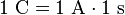# Coulomb facts for kids

Kids Encyclopedia Facts

The coulomb (sometimes written C) is the SI unit of electric charge. It is named after Charles-Augustin de Coulomb.

A coulomb is a quantity of electric charge. The movement of electric charge is called current in an electric circuit. For example, a flashlight bulb that has a current of one ampere will pass one coulomb of charge every second. So in ten seconds, ten coulombs have passed through the light bulb.

In an electric wire the charge that moves is in a particle called the electron. Each electron has a very small electric charge of negative one elementary charge. The elementary charge is the smallest electric charge found in a stable particle. A coulomb is equal to 6,241,509,629,152,650,000 elementary charges. The coulomb was named in honor of Charles-Augustin de Coulomb who worked on understanding electric charge in the late 1700s.

Technical Definition:

1 coulomb is the amount of electric charge carried by a current of 1 ampere flowing for 1 second.$1 \ \mathrm{C} = 1 \ \mathrm{A} \cdot 1 \ \mathrm{s}$Coulomb Facts for Kids. Kiddle Encyclopedia.